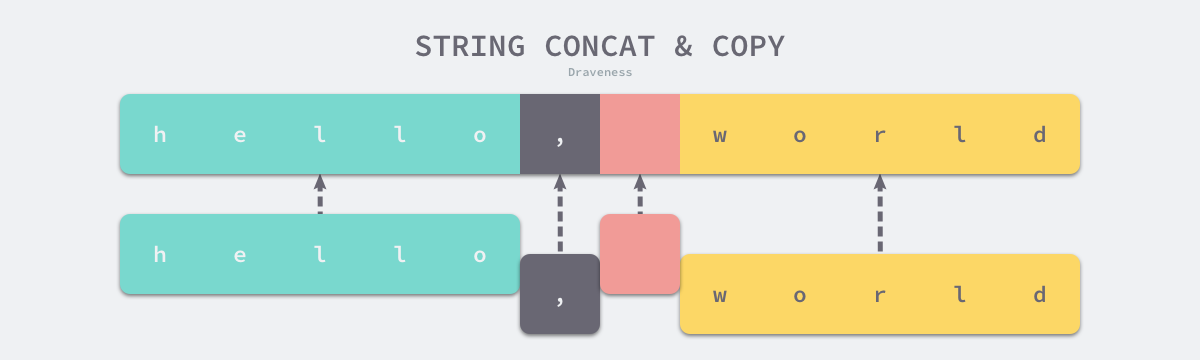Go 语言字符串和字节数组的实现原理

3.4 字符串 #$cat main.go package main func main() { str := "hello" println([]byte(str)) }$ GOOS=linux GOARCH=amd64 go tool compile -S main.go
...
go.string."hello" SRODATA dupok size=5
0x0000 68 65 6c 6c 6f                                   hello
...

1. 先将这段内存拷贝到堆或者栈上；
2. 将变量的类型转换成 []byte 后并修改字节数据；
3. 将修改后的字节数组转换回 string

Java、Python 以及很多编程语言的字符串也都是不可变的，这种不可变的特性可以保证我们不会引用到意外发生改变的值，而因为 Go 语言的字符串可以作为哈希的键，所以如果哈希的键是可变的，不仅会增加哈希实现的复杂度，还可能会影响哈希的比较。

Data uintptr
Len  int
}

Data uintptr
Len  int
Cap  int
}

3.4.2 解析过程 #

str1 := "this is a string"
str2 := this is another
string

json := {"author": "draven", "tags": ["golang"]}

func (s *scanner) stdString() {
s.startLit()
for {
r := s.getr()
if r == '"' {
break
}
if r == '\\' {
s.escape('"')
continue
}
if r == '\n' {
s.ungetr()
s.error("newline in string")
break
}
if r < 0 {
s.errh(s.line, s.col, "string not terminated")
break
}
}
s.nlsemi = true
s.lit = string(s.stopLit())
s.kind = StringLit
s.tok = _Literal
}

1. 标准字符串使用双引号表示开头和结尾；
2. 标准字符串需要使用反斜杠 \ 来逃逸双引号；
3. 标准字符串不能出现如下所示的隐式换行 \n
str := "start
end"

func (s *scanner) rawString() {
s.startLit()
for {
r := s.getr()
if r == '' {
break
}
if r < 0 {
s.errh(s.line, s.col, "string not terminated")
break
}
}
s.nlsemi = true
s.lit = string(s.stopLit())
s.kind = StringLit
s.tok = _Literal
}

func (p *noder) basicLit(lit *syntax.BasicLit) Val {
switch s := lit.Value; lit.Kind {
case syntax.StringLit:
if len(s) > 0 && s == '' {
s = strings.Replace(s, "\r", "", -1)
}
u, _ := strconv.Unquote(s)
return Val{U: u}
}
}

strconv.Unquote 处理了很多边界条件导致实现非常复杂，其中不仅包括引号，还包括 UTF-8 等编码的处理逻辑，这里也就不展开介绍了。

3.4.3 拼接 #

func walkexpr(n *Node, init *Nodes) *Node {
switch n.Op {
...
}
}

• 如果小于或者等于 5 个，那么会调用 concatstring{2,3,4,5} 等一系列函数；
• 如果超过 5 个，那么会选择 runtime.concatstrings 传入一个数组切片；
func addstr(n *Node, init *Nodes) *Node {
c := n.List.Len()

buf := nodnil()
args := []*Node{buf}
for _, n2 := range n.List.Slice() {
args = append(args, conv(n2, types.Types[TSTRING]))
}

var fn string
if c <= 5 {
fn = fmt.Sprintf("concatstring%d", c)
} else {
fn = "concatstrings"

t := types.NewSlice(types.Types[TSTRING])
slice := nod(OCOMPLIT, nil, typenod(t))
slice.List.Set(args[1:])
args = []*Node{buf, slice}
}

cat := syslook(fn)
r := nod(OCALL, cat, nil)
r.List.Set(args)
...

return r
}

func concatstrings(buf *tmpBuf, a []string) string {
idx := 0
l := 0
count := 0
for i, x := range a {
n := len(x)
if n == 0 {
continue
}
l += n
count++
idx = i
}
if count == 0 {
return ""
}
if count == 1 && (buf != nil || !stringDataOnStack(a[idx])) {
return a[idx]
}
s, b := rawstringtmp(buf, l)
for _, x := range a {
copy(b, x)
b = b[len(x):]
}
return s
}3.4.4 类型转换 #

func slicebytetostring(buf *tmpBuf, b []byte) (str string) {
l := len(b)
if l == 0 {
return ""
}
if l == 1 {
stringStructOf(&str).str = unsafe.Pointer(&staticbytes[b])
stringStructOf(&str).len = 1
return
}
var p unsafe.Pointer
if buf != nil && len(b) <= len(buf) {
p = unsafe.Pointer(buf)
} else {
p = mallocgc(uintptr(len(b)), nil, false)
}
stringStructOf(&str).str = p
stringStructOf(&str).len = len(b)
memmove(p, (*(*slice)(unsafe.Pointer(&b))).array, uintptr(len(b)))
return
}

func stringtoslicebyte(buf *tmpBuf, s string) []byte {
var b []byte
if buf != nil && len(s) <= len(buf) {
*buf = tmpBuf{}
b = buf[:len(s)]
} else {
b = rawbyteslice(len(s))
}
copy(b, s)
return b
}

• 当传入缓冲区时，它会使用传入的缓冲区存储 []byte
• 当没有传入缓冲区时，运行时会调用 runtime.rawbyteslice 创建新的字节切片并将字符串中的内容拷贝过去；3.4.6 延伸阅读 #

1. 火焰图是一种分析程序性能的手段，Flame Graphs http://www.brendangregg.com/flamegraphs.html ↩︎